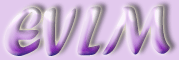Expectation

Target: On completion of this worksheet you should understand and be able to calculate the expected value for a variable.

Suppose a fair coin is tossed 100 times. How many times would we expect to get a head? Common sense tells us that we would expect to get heads about 50 times. We calculate:

Expected no. of heads =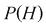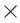100.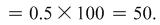We know that we will not get exactly 50 heads but this is the average called the expected value and is written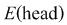.

## 1.1  Examples

1. A manufacturing process produces 3% defective items. These items are packed in boxes of 500. How many defective items would you expect?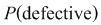= 0.03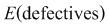= 0.03500 = 15.

2. A game costs 20p a go. The rules are: throw a fair dice and you win 50p if the score is 3 or more. The game is played 300 times. How much are you likely to win or lose overall and what is the profit or loss per go?

P(win)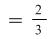.

Cost for 300 attempts is 30020p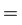£60

Expected number of wins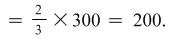Expected amount won = 20050p = £100

Expected profit£100 - £60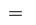£40.

Profit per go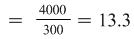p.

This profit does not depend on the number of times the game is played and we can calculate it by considering the profit per go (loss is shown by negative amount):

 Score 1 or 2 3, 4, 5, 6 Probability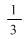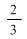Profit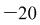p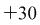p

If we call the profit X then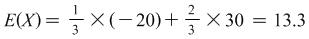p as above.

3. A company manufactures computer parts. After tests a part is classified as A or B or rejected. The probability of classification A is 0.7 and of B is 0.25. The company makes a profit of 60p for an A part, 40p for a B part and a loss of 10p if the part is rejected. Find the expected profit per part.

P(defective) = 1 – 0.7 – 0.250.05.

We will put the information into a table:

 Type A B Reject Probability 0.7 0.25 0.05 Profit 60p 40p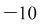p

Let the profit be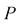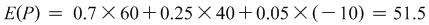p